### RhombusEvery rhombus is a parallelogram, and a rhombus with right angles is a square. ==Etymology== The word `rhombus` comes from Greek ῥόμβος(rhombos), meaning something that spins, which derives from the verb ρέμβω (rhembō), meaning `to turn round and round`. The word was used both by Euclid and Archimedes, who used the term `solid rh...
Found on http://en.wikipedia.org/wiki/Rhombus

### Rhombus• (n.) Same as Rhomb, 1.
Found on http://thinkexist.com/dictionary/meaning/rhombus/

### rhombusrhomb noun a parallelogram with four equal sides; an oblique-angled equilateral parallelogram
Found on https://www.encyclo.co.uk/local/20974

### RhombusRhom'bus noun [ Latin ] Same as Rhomb , 1.
Found on http://www.encyclo.co.uk/webster/R/78

### rhombus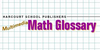A parallelogram with four congruent sides
Example: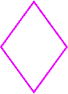Found on http://www.hbschool.com/glossary/math2/index6.html

### RhombusA parallelogram with four equal sides.
Found on http://www.diracdelta.co.uk/science/source/r/h/rhombus/source.html

### rhombusA quadrilateral in which both pairs of opposite sides are parallel and all sides are the same length, that is, an equilateral parallelogram. A rhombus is also sometimes called a rhomb or a diamond. A rhombus whose acute angles are 45° is called a lozenge. The diagonals p and q of a rhombus are perpe...
Found on http://www.daviddarling.info/encyclopedia/R/rhombus.html

### Rhombus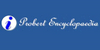A rhombus or rhomb is a parallelogram having its four sides equal and its opposite sides equal, but with two opposite angles acute and two obtuse.
Found on http://www.probertencyclopaedia.com/browse/GR.HTM

### rhombus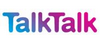Equilateral (all sides equal) parallelogram. As with a parallelogram, the rhombus has diagonally opposed angles of equal size. Its diagonals bisect each other at right angles, and its area is half the product of the lengths of the two diagonals. The shape is sometimes called a diamond. A rhombus whos...
Found on http://www.talktalk.co.uk/reference/encyclopaedia/hutchinson/m0006730.html

### rhombus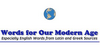rhombus (s), rhombi (pl) 1. A parallelogram that has four equal sides and oblique angles. 2. A parallelogram with four equal sides and sometimes one with no right angles.
Found on http://www.wordinfo.info/words/index/info/view_unit/1853/

### rhombus[n] - a parallelogram with four equal sides
Found on http://www.webdictionary.co.uk/definition.php?query=rhombus
No exact match found